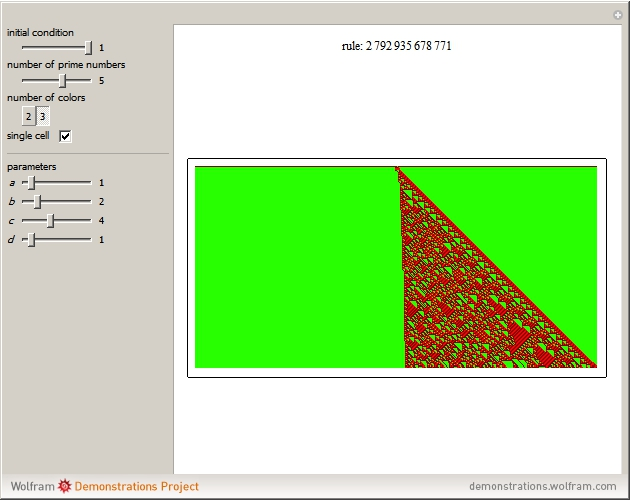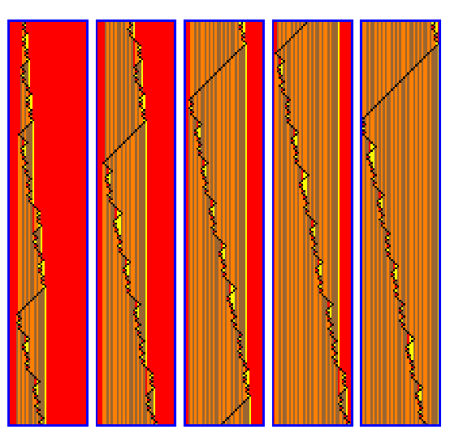#Wolfram Summer School

# Alumni# André Brasil Cavalcante

Summer School

Class of 2012

## Bio

André Brasil Cavalcante did his undergraduate studies in civil engineering at University of Brasilia and received his PhD in geotechnics from University of Brasilia in 2004. Since 2010, he has held a professor position at the postgraduate program in geotechnics from University of Brasilia. He is interested in modeling physical phenomena such as water and solute flow through soil using cellular automata by means of the universal iota-delta function.

## Project: Using the Universal Iota-Delta Function for Cellular Automata Analysis of Physical Phenomenon

Iota-delta function is a universal function, discovered by me and my research students Luan Ozelim and Lucas Borges, that is a numerical function that calculates all cellular automata. Because it is a numerical function, we can use it to generalize discrete cellular automata to continuous problems. Also, we have developed a numerical scheme for continuous problems using iota-delta, and for this project, we are going to clarify these ideas and explore the generalization of the discrete to the continuous.

1. We are going to give formal and Mathematica definitions for the iota-delta and iota-delta functions.
2. We are going to look for some combinations that could generate some special rules; in particular, we are going find all ways for getting the elementary cellular automata with small primes.
3. We are going to verify for different initial conditions if the rule could generate the same pattern.
4. We are going to search for the smallest prime number that could generate all three-color automata.
5. We are going to implement the iota-delta numerical method and prove that some rules could perform the finite difference method.
6. We are going to prove that finite difference method is a particular case of iota-delta numerical method.
7. We are also investigating other numerical cases based on the cellular automata.
8. And finally, if given God’s help, we will find the Turing’s machine that could generate iota-delta.

## Project-Related Demonstrations

### Iota-Delta Function for Generating Cellular Automata## Favorite Four-Color, Four State Turing Machine

Rule 382784415773470871062475• 21st Annual Wolfram Summer School
• Bentley University, Waltham, MA, USA
• June 25–July 15, 2023